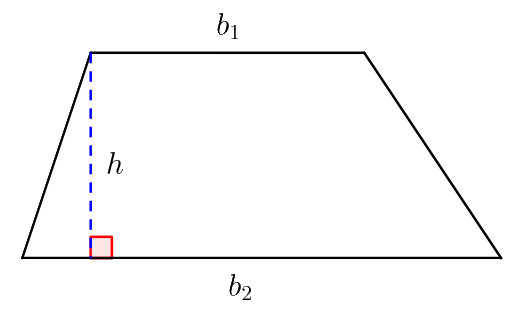# CAT 2021 Set-2 | Quantitative Aptitude | Question: 8

1 vote
70 views

The sides $\text{AB}$ and $\text{CD}$ of a trapezium $\text{ABCD}$ are parallel, with $\text{AB}$ being the smaller side. $\text{P}$ is the midpoint of $\text{CD}$ and $\text{ABPD}$ is a parallelogram. If the difference between the areas of the parallelogram $\text{ABPD}$ and the triangle $\text{BPC}$ is $10 \; \text{sq cm},$ then the area, $\text{in sq cm},$ of the trapezium $\text{ABCD}$  is

1. $25$
2. $30$
3. $40$
4. $20$

retagged

1 vote

Let’s draw the trapezium for better understanding.Let $\text{PD} = a \; \text{cm} \Rightarrow \text{PC} = a \; \text{cm} \Rightarrow \text{CD} = 2a \; \text{cm}$

Area of the parallelogram $\text{ABPD}= a \times h \; \text{cm}^{2}$

Area of $\triangle \text{BPC} = \frac{1}{2} \times a \times h \; \text{cm}^{2}$

Now, $ah – \frac{1}{2}ah = 10$

$\Rightarrow 2ah – ah = 20$

$\Rightarrow \boxed{ah = 20 \; \text{cm}^{2}}$

$\therefore$ Area of the trapezium $\text{ABCD} = \frac{1}{2} (a + 2a) \times h$

$\qquad \qquad = \frac{1}{2} \times 3ah = \frac{1}{2} \times 3(20) = 30 \; \text{cm}^{2}.$

Correct Answer $: \text{B}$

$\textbf{PS:}$ A trapezium, also known as a trapezoid, is a quadrilateral in which a pair of sides are parallel, but the other pair of opposite sides are non-parallel. The area of a trapezium is computed with the following formula:

$$\text{Area}=\frac {1}{2} × \text {Sum of parallel sides} × \text{Distance between them}.$$The parallel sides are called the bases of the trapezium. Let $b_1$ and $b_2$​ be the lengths of these bases. The distance between the bases is called the height of the trapezium. Let $h$ be this height. Then this formula becomes:

$$\boxed{\text{Area}=\frac{1}{2}(b_1+b_2)h}$$

10.1k points 4 8 30
edited

## Related questions

1 vote
1
81 views
If a rhombus has area $12 \; \text{sq cm}$ and side length $5 \; \text{cm},$ then the length, $\text{in cm},$ of its longer diagonal is $\sqrt{13} + \sqrt{12}$ $\sqrt{37} + \sqrt{13}$ $\frac{\sqrt{37} + \sqrt{13}}{2}$ $\frac{\sqrt{13} + \sqrt{12}}{2}$
1 vote
2
84 views
Let $\text{D}$ and $\text{E}$ be points on sides $\text{AB}$ and $\text{AC},$ respectively, of a triangle $\text{ABC},$ such that $\text{AD}$ : $\text{BD} = 2 : 1$ and $\text{AE}$ : $\text{CE} = 2 : 3.$ If the area of the triangle $\text{ADE}$ is $8 \; \text{sq cm},$ then the area of the triangle $\text{ABC, in sq cm},$ is
1 vote
Consider the pair of equations: $x^{2} – xy – x = 22$ and $y^{2} – xy + y = 34.$ If $x>y,$ then $x – y$ equals $7$ $8$ $6$ $4$
Anil, Bobby and Chintu jointly invest in a business and agree to share the overall profit in proportion to their investments. Anil's share of investment is $70 \%.$ His share of profit decreases by $₹ \; 420$ if the overall profit goes down from $18 \%$ to $15 \%.$ Chintu's share of ... goes up from $15 \%$ to $17 \%.$ The amount, $\text{in INR},$ invested by Bobby is $2400$ $2200$ $2000$ $1800$
The number of ways of distributing $15$ identical balloons, $6$ identical pencils and $3$ identical erasers among $3$ children, such that each child gets at least four balloons and one pencil, is